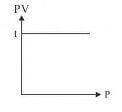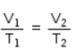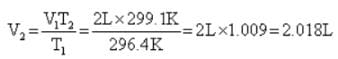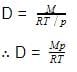NEET  >  Test: The Gas Laws

# Test: The Gas Laws - NEET

Test Description

## 15 Questions MCQ Test Chemistry Class 11 - Test: The Gas Laws

Test: The Gas Laws for NEET 2023 is part of Chemistry Class 11 preparation. The Test: The Gas Laws questions and answers have been prepared according to the NEET exam syllabus.The Test: The Gas Laws MCQs are made for NEET 2023 Exam. Find important definitions, questions, notes, meanings, examples, exercises, MCQs and online tests for Test: The Gas Laws below.
Solutions of Test: The Gas Laws questions in English are available as part of our Chemistry Class 11 for NEET & Test: The Gas Laws solutions in Hindi for Chemistry Class 11 course. Download more important topics, notes, lectures and mock test series for NEET Exam by signing up for free. Attempt Test: The Gas Laws | 15 questions in 15 minutes | Mock test for NEET preparation | Free important questions MCQ to study Chemistry Class 11 for NEET Exam | Download free PDF with solutions
 1 Crore+ students have signed up on EduRev. Have you?
Test: The Gas Laws - Question 1

### The slope of plot between pV and p at constant temperature is:

Detailed Solution for Test: The Gas Laws - Question 1

Slope of the plot between P and PV at constant temperature is zero. A plot of P v/s PV at constant temperature for a fixed mass of gas is a straight line parallel to the pressure axis.Test: The Gas Laws - Question 2

### Mathematical expression that describes Boyle's law is

Test: The Gas Laws - Question 3

### At constant temperature, the pressure of a fixed amount of gas varies inversely with its volume, is

Detailed Solution for Test: The Gas Laws - Question 3

The correct answer is option A.
Boyle's law:
In 1662 Robert Boyle studied the relationship between volume and pressure of a gas of fixed amount at constant temperature. He observed that volume of a given mass of a gas is inversely proportional to its pressure at a constant temperature.

Test: The Gas Laws - Question 4

Dalton’s law of partial pressure states that

Detailed Solution for Test: The Gas Laws - Question 4

The correct answer is option B
Dalton's law (also called Dalton's law of partial pressures) states that in a mixture of non-reacting gases, the total pressure exerted is equal to the sum of the partial pressures of the individual gases. This empirical law was observed by John Dalton in 1801 and published in 1802.

Test: The Gas Laws - Question 5

At constant volume, pressure of a fixed amount of a gas varies directly with the temperature, is

Detailed Solution for Test: The Gas Laws - Question 5

Gay Lussac’s law states that the pressure of a fixed mass of a gas at constant volume is directly proportional to the temperature on Kelvin scale.

Test: The Gas Laws - Question 6

The vapour pressure of water at 300 K in a closed container is 0.4 atm. If the volume of the container is doubled, its vapour pressure at 300 K will be

Detailed Solution for Test: The Gas Laws - Question 6

The vapour pressure of water will remain the same as the temperature is unchanged. So, the answer is c) 0.4 atm

Test: The Gas Laws - Question 7

The value of universal gas constant R depends on:

Detailed Solution for Test: The Gas Laws - Question 7

We have

R = PV/T

= 8.314JK−1mol−1

= 8.314x107ergK−1mol−1

= 2calK−11mol−1

= 0.0821litreatmK−1mol−1

Hence R depends on units of volume and pressure.

Test: The Gas Laws - Question 8

Equal volumes of all gases under the same conditions of temperature and pressure contain equal number of molecules, is

Detailed Solution for Test: The Gas Laws - Question 8

Avogadro's law states that, "equal volumes of all gases, at the same temperature and pressure, have the same number of molecules."

Test: The Gas Laws - Question 9

Use of hot air balloons is an application of:

Detailed Solution for Test: The Gas Laws - Question 9

The relationship between the temperature and volume of a gas, which is known as Charles' law, provides an explanation of how hot-air balloons work.

Test: The Gas Laws - Question 10

A vessel contains 0.16g of methane and 0.28g of nitrogen. The ratio of partial pressures of methane to nitrogen in the mixture is about:

Detailed Solution for Test: The Gas Laws - Question 10

We know, Partial pressure of a gas = mole fraction of that gas x Total pressure of mixture of the gases.

Using this formula and using the information given in this question, we get
ratio of partial pressures of methane to nitrogen in the mixture as: 1:1

Test: The Gas Laws - Question 11

A balloon seller filled a balloon with 2 L air when the temperature was 23.4° C. He sold the balloon at the time when temperature was recorded to 26.1°C. Volume of the balloon at this temperature is

Detailed Solution for Test: The Gas Laws - Question 11

From Charles law we conclude thatTest: The Gas Laws - Question 12

Graph for Boyle's law is called

Detailed Solution for Test: The Gas Laws - Question 12

For Boyle’s law, temperature is constant. So the graph is called as isotherm.

Test: The Gas Laws - Question 13

One mole of N2O4 is kept in a close container at 300K and 1 atm pressure. When it is heated to 600 K 20% of it decomposes to NO2 (g). Calculate the resultant pressure exerted on the walls of the container

Detailed Solution for Test: The Gas Laws - Question 13

N2O⇔ 2NO2
1mole 0 Initial moles
-20% of 1=0.2  +2(20% of 1)=0.40  At equilibrium
1-0.2= 0.8  0.4  Moles at equilibrium
Total moles at equilibrium = 0.8+0.4 = 1.2 moles
1 mole vapour pressure = 1 atm at 300 K
Applying PV = nRT
1xV = 1x R x 300 .... (1)
When n=1.2 moles, T = 600 K
P x V = 1.2 x R x 600 .... (2)
Dividing (2) by (1),
PxV/(1xV) = (1.2 x R x 600)/ (1 x R x 300)
Therefore, P= 2.4 atm
Hence, resultant pressure of mixture is 2.4 atm

Test: The Gas Laws - Question 14

The density of the gas is equal to:

Detailed Solution for Test: The Gas Laws - Question 14

The correct answer is Option C.
For ideal gas
pV=RT
V=RT/p
Density is given by
D=M/V
Where M is mass and V is volume
Putting the value of VTest: The Gas Laws - Question 15

For a certain reaction PV = 2 dm3 atm and volume is 4 dm3 then corresponding pressure is

Detailed Solution for Test: The Gas Laws - Question 15

P1V1=P2V2
2=P2×4
P2=0.5

## Chemistry Class 11

199 videos|339 docs|229 tests
Information about Test: The Gas Laws Page
In this test you can find the Exam questions for Test: The Gas Laws solved & explained in the simplest way possible. Besides giving Questions and answers for Test: The Gas Laws, EduRev gives you an ample number of Online tests for practice

## Chemistry Class 11

199 videos|339 docs|229 tests

### How to Prepare for NEET

Read our guide to prepare for NEET which is created by Toppers & the best Teachers(Scan QR code)